# Kerala Syllabus 9th Standard Maths Solutions Chapter 10 Real Numbers

## Kerala State Syllabus 9th Standard Maths Solutions Chapter 10 Real Numbers

### Real Numbers Textual Questions and Answers

Textbook Page No. 160

Question 1.
Find the distance between the two points on the number line, denoted by each pair of numbers given below: .Question 2.
Find the midpoint of each pair of points in the first problem.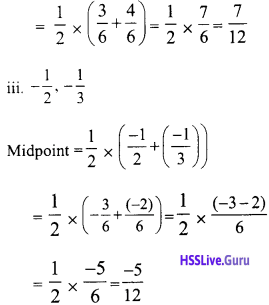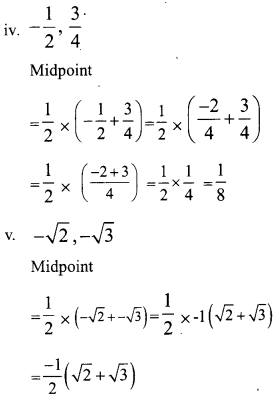Question 3.
The part of the number line between the points denoted by the numbers 1/3 and 1/2 is divided into four equal parts. Find the numbers de-noting the ends of each such part.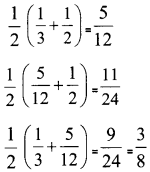So the portion between 1/3 and 1/2 is divided into 4 equal parts the points areTextbook Page No. 164

Question 1.
Find those x satisfying each of the equations below:
i. |x – 1| = |x – 3|
ii. |x – 3| = |x – 4|
iii. |x + 2| = |x – 5|
iv. |x| = |x + 1|
i. $$|x-1|=|x-3|$$
$$|x-1|$$ means distance between x and 1
$$|x-3|$$ means distance between x and 3. Therefore the distance from x to 1 and 3 are equal.
x is in between 1 and 3 , that is x is the midpoint of 1 and 3.
$$x=\frac{1}{2} \times(1+3)=\frac{1}{2} \times 4=2$$

ii. $$|x-3|=|x-4|$$
The distance from x to 3 and 4 are equal.
∴ x is in between 3 and 4 , that is x is the midpoint of 3 and 4.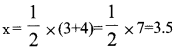iii. $$\begin{array}{l}{|x+2|=|x-5|} \\ {|x+2|=|x-(-2)|}\end{array}$$
The distance from x to -2 and 5 are equal.
∴ x is in between -2 and 5 , that is x is the midpoint of -2 and 5.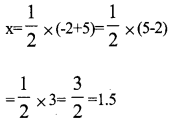iv. |x| = |x + 1|

|x + l] = ]x – (-l)|
The distance from x to -1 and 0 are equal.
∴ x is in between -1 and 0, that is x is the midpoint of -1 and 0.Question 2.
Prove that if 1 < x < 4 and 1 < y < 4, then |x – y| < 3.
1 < x < 4 , possible values of x = 2, 3 1 possible values of y = 2, 3
|x – y| = |2 – 2| = 0 < 3
|x – y| = |3 – 2| – 1 < 3
|x – y| = |2 – 3| = |-l| = l < 3
|x – y| = |3 – 3| = 0 < 3
From this if 1 < x < 4, 1 < y < 4, then |x – y| < 3.

Question 3.
Prove that if x < 3 and y > 7, then |x – y) > 4 .
Since x < 3, the maximum value of x is less than 3. Since y > 7 the minimum value of y is greater than 7.
Then the difference between the minimum values of y and the maximum value of x is greater than 7 – 3 = 4.
i.e., |x – y| > 4Question 4.
Find two numbers x, y such that
$$|x+y|=|x|+|y|$$
1. If x = 3, y =7, then
| x + y | =  |13 + 71| = 3 + 7 = 10
|x| + |y| = |3| + |7| = 3 + 7 = 10
|x + y| = |x| + |y|

2. If x = -6, y =-9 , then
|x + y| = |-6 + -9|
= l-15| = 15

Question 5.
Are there numbers x,y such that |x + y| < |x| + |y| ?
x = 5, y = -3

|x+y| = |5 + -3| = |5 – 3| = |2| = 2

|x| = |5| = 5

|y| = |-3| = 3 |x| + |y| = 5 + 3 = 8

Among x, y, if one is a positive number and the other is a negative number, then
| x + y | < |x| + |y|. Question 6. Are there numbers x, y such that |x + y| > |x| + |y| ?
No

Question 7.
What are the numbers x, for which
|x – 2| + |x – 8| = 6
$$|x-2|$$ means distance between x and 2.
x can be to the right or left side of the number 2.
$$|x-8|$$ means distance between x and 8
x can be to the right or left side of the number 8.
If we add the distance between x and 2 with x and 8 we get 6.
When x is to the left side of 2, when we add the distance of x to 2 and 8 we get a number which is greater than 6.
Distance between 2 and 8
= |2 – 8| = | -6| = 6
The difference between 2 and 8 is 6, so the position of x is between 2 and 8.
That is the value of x is in between 2 and 8 including 2 and 8.
i. e., 2 ≤ x ≤ 8

Question 8.
What are the numbers x, for which
|x – 2| + |x – 8| = 10 ?
Taking x as different numbers, what all numbers do we get as
|x – 2| + |x – 8| ?
|x – 2| + |x – 8| = 10, |x – 2| – |x – 8| = 10
x – 2 + x – 8 = 10
x = 10
( x – 2) – (x – 8) = 10
There is no number x which satisfies this.
– ( x- 2) + (x – 8) = 10
There is no number x which satisf¬ies this.
-(x – 2) – (x – 8) =10
-x + 2 – x + 8=10
-2x = 0
= x = 0
i.e., x = 0, 10

If x = 1
|x – 2| + |x – 8| = |1 – 2| + |1 – 8|
= | -1 | + | -7| = 1 + 7 = 8

If x = 2
|x – 2| + |x – 8| = |2 – 2| + |2 – 8|
= |0| + | -6| = 0 + 6 = 6

If x = 3
|x – 2| + |x – 8| = |3 – 2| + |3 – 8|
= | -1| + | -5| = 1 + 5 = 6

If x = 4
|x – 2| + |x – 8| = |4 – 2| + |4 – 8|
= | -2| + | -4| = 2 + 4 = 6

If x = 5
|x – 2| + |x – 8| = [5 – 2| +15 – 8|
= | -3| + | -3| = 3 + 3 = 6

If x=6
|x – 2| + |x – 8| = |6 – 2| + |6 – 8|
= | -4|+ | -2| = 4 + 2 = 6

If x = 7
|x – 2| + |x – 8| = |7 – 2| + |17 – 8|
= |-5| + |-1| = 5 + 1 = 6
i.e., |x – 2| + |x – 8| = 6 when 2 < x < 8

If x=9
|x – 2| + |x – 8| = |9 – 2| + |9 – 8|
= |7| + |1| = 7 + 1 = 8

If x= 11
|x – 2| + |x – 8| = |11 – 2| + |11 – 8|
= |9| + |3|= 9 + 3 = 12
…………. etc
Taking x as different numbers, different numbers get as |x – 2| + |x – 8|.

### Real Numbers Exam Oriented Questions and Answer

Question 1.
a. Which number indicates the point located 7 units to left of 5 on the number line?
b. Write down the numbers located 2 units apart from these points.
a. The point which is 7 units to the left of 5 = 5 – 7 = -2
b. There is one point 2 unit apart to the left and right side of -2 .
The point which is 2 units to the left of -2 = -4
The point which is 2 units to the right of -2 = 0

Question 2.
How many numbers are on the number line which are 11 units apart from 5. What are they ? Calculate the distance between the numbers.
There is one point 11 unit apart to the left and right side of 5.
Point on the left side = 5 + -11 = -6
Point on the right side = 5 + 11 = 16
Distance between them = 16 – (-6) = 16 + 6 = 22 unit

Question 3.
Find the distance between the num-bers given below on the number line.
i. 4, 6
ii. 3, -2
iii. -5, -8
iv. 3/5, 5/6
i. Distance = |6 – 4| = |2| = 2

ii. Distance = |3 – (-2)| = |3 + 2| = |5| = 5

iii. Distance = |-5 -(-8)| =|-5 + 8|
= |+3| = 3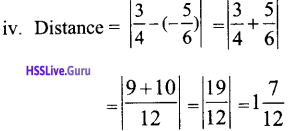Question 4.
The endpoints of one side of an equilateral triangle are -2 and 4 on the number line. Calculate the area and perimeter of the triangle.
The distance between -2 and 4 = 4 – (-2) = 6
One side of a equilateral triangle = 6 unit Perimeter of an equilateral triangle
= 3 × 6 = 18 unit Area of an equilateral triangleQuestion 5.
The number x is on the number line, then find x if |x – 10| = – |x – 4|.
The distance between x to 10 and 4 are same. Therefore x is the midpoint of 4 and 10.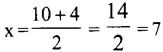Question 6.
The quadrilateral ABCD is a square. A and B the points denote -2 and 3 on the number line.
a. Draw the square ABCD on the number line
b. Calculate the perimeter of the square ABCD.b. AB = |-2 – 3| = 5 unit
Perimeter = 5 × 4 = 20 unit

Question 7.
If |a + 1| = |a + 5 |, |b – 2| = |b – 6|,
|a – x| = |b -x|, then find x.
|a + 1| = |a + 5|
Since the point, ‘a’ represented on the num¬ber line is at a the equal distance from -1 and -5.
That is ‘a’ is midpoint of -1 and -5.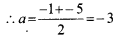|b – 2| = |b – 6|
Since the point, ‘a’ represented on the number line is at a equal distance from 2 and 6.
That is ‘a’ is midpoint of 2 and 6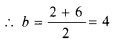|x – a| = |x – b| therefore point x is the
midpoint of a = -3 and b = 4Question 8.
Find the value of x.
a. |x – 2| = |x – 10|
b. |x + 3| = |x – 7|
a. The distance between x to 2 and 10 are same. Therefore x is the midpoint of 2 and 10.b. |x + 3| = |x – (-3)|
The distance between x to -3 and 7 are same. Therefore x is the midpoint of -3 and 7.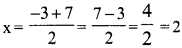Question 9.
If the difference between two numbers is 4. If one number is 8. What values will the other number have?
Consider other number as x, then
|x – 8| = 4
i.e., x – 8 = 4 or 8 – x = 4
x = 12 or x = 4
Other number as 12 or 4.

Question 10.
Find the distance between the pairs of numbers given below.
a. 2, 8
b. -2, 8
c. -2, -8
a. Distance between 2 and 8
= |x – y| = |2 – 8| |-6| = 6

b. Distance between -2 and 8
= |-2-8| = |-10| = 10

c. Distance between -2 and -8
= |-2 – (-8)| = |-2 + 8| = |6| = 6

Question 11.
Draw the number line. Mark the position of numbers given below.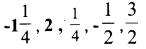Question 12.
The number x is located to the right side of 3 on the number line, if
|x – 3|= 0, then
a. What is the value of x ?
b. Calculate |x – 3| + |x – 9|.
a. |x – 3| = 0
-(x – 3) = 0 or x – 3 = 0
-x = -3 or x = 3
x=3

b. |x – 3| + |x – 9|
= |3 – 3| + |3 – 9|
= |0| + |-6 | = 6

Question 13.
If |x – 5| = 10, then find the value of x .
The distance between x and 5 is 10.
If x is to the right side of 5 , then x = 5 + 10 = 10
If x is to the left side of 5 , then
x = 5 – 10 = -5

Question 14.
If |x + 2| = |x – 8|, then find the value of x ?
|x + 2| = |x – (-2)|
The distance of x to -2 and 8 are equal.
Therefore position of x is between -2 and 8.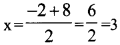Question 15.
If |x – 1| =|x – 3|, then find the value of x.
Distance from 1 and 3 to x are same.
Therefore position of x is between 1 and 3.
Position of x = $$\frac { 1 + 3 }{ 2 }$$ = $$\frac { 4 }{ 2 }$$ = 2

Question 16.
Using the common form of rational numbers, prove that the sum, difference, product and quotient of any two rational numbers is again a rational number.
i. If a, b, c and d are natural numbers,
$$\frac { a }{ b }$$, $$\frac { c }{ d }$$ are rational numbers.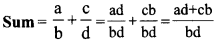Sum and product of all-natural numbers are always natural numbers. So here the numerator and denominator are natural numbers.
That is if x and y are natural numbers
then this can be expressed as x/y.
Therefore the sum is rational

ii. Difference:
a, b, c and d are natural numbersHence it is rational.

iii. Product:
a, b, c and d are natural numbers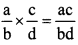Hence it is rational.

iv. Quotient:
a, b, c and d are natural numbers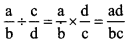Hence it is rational.Question 17.
Prove that the product of any irrational number and non – zero rational number is an irrational number.
Let a be the irrational number and b the rational number and a × b = c, that is to prove that c is irrational.
Consider c is a rational number, a × b = c
From this we get b = c/a
That is b = $$\frac { One rational }{ other rational }$$ = One rational
If we take b as irrational here we get b as a rational number. This is a wrong decision. This is due to our wrong assumption that is c is a rational number. The assumption that c is a rational number is a wrong one.
∴ c is an irrational number.
That is the product of any irrational number and non – zero rational number is an irrational number.

Question 18.
Give an example of two different irrational numbers whose product is a rational number.